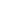科研动态最新资讯

• 天元讲堂（11.29）​The Kozlov completeness problem
• 浏览量：116 发布人： 发布时间：2019-11-28

• 报告题目：The Kozlov completeness problem

报告人：郭坤宇教授(复旦大学)

时间：11月29日下午3：30--4：30

地点：数学楼410

摘要  ：The classical completeness problem raised by Beurling(1945) and independently by Wintner(1944-1945) asks for which $\psi\in L^2(0,1)$, the  dilation system $\{\psi(kx):k=1,2,\cdots\}$ is complete in $L^2(0,1)$, where $\psi$ is identified with its odd $2$-periodic extension  on $\mathbb{R}$. This difficult problem is nowadays commonly called as the Periodic Dilation Completeness Problem (PDCP). The PDCP  has a natural link  with the famous Riemann Hypothesis. Since the set of simple functions on $(0,1)$ is dense in $L^2(0,1)$, ones  focus on   the above problem for the class of characteristic functions. Let $\chi_s$ be the characteristic function of $(0,s)$, $0<s\leq 1$, and $\mathcal{D}_s=\{\chi_s(kx):k=1,2,\cdots\}$.  The Kozlov completeness problem is  to  ask for which $s$, the  dilation system $\mathcal{D}_s$ is complete(1948-1950). In this talk, I will give a brief introduction for what we have made some  significant progress on the PDCP and the  Kozlov  problem. This is a joint work with Dr.Dan.

简介：郭坤宇，复旦大学数学科学学院教授、博导。复旦大学“非线性数学模型与方法”教育部重点实验室主任,复旦大学数学科学学院学术委员会主任。国家杰出青年科学基金获得者、教育部长江学者特聘教授。曾任复旦大学数学科学学院院长, 宁夏教育厅副厅长（挂职）。